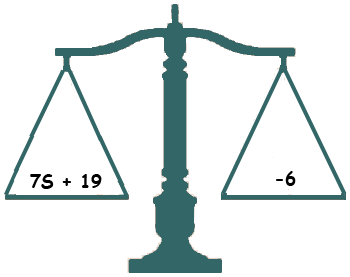Subject: MATH Name: Eriona Who are you: Student (Middle) 6p-5=17. I have to solve the equation. Eriona, I am going to solve a similar but different question. Solve 7s + 19 = -6 I find that it helps to think of such an equation as a balance, the kind you might see in a physics lab with two pans that balance.On the left pan is the left side of the equation, 7s + 19 and on the right pan is the right side of the equation, - 6. The balance between them is the equal sign. (It might seem strange to have a negative weight, but it's only an analogy.) The task is to manipulate the weights, always maintaining a balance, to the place where you have s alone one one pan and a number on the other, then you know that s and that number have the same value, that is they are equal. The balance will be maintained if I add a weight of -19 to both sides. Algebraically this is 7s + 19 = - 6 and thus 7s + 19 - 19 = - 6 - 19 which is 7s = - 25 At this point you are almost done. You want s alone on the left side and you have 7 times s. The balance will be maintained if you divide both sides by 7 and hence 7s = - 25 and thus 7s/7 = - 25/7 so s = -3 4/7 Now try your equation. Penny